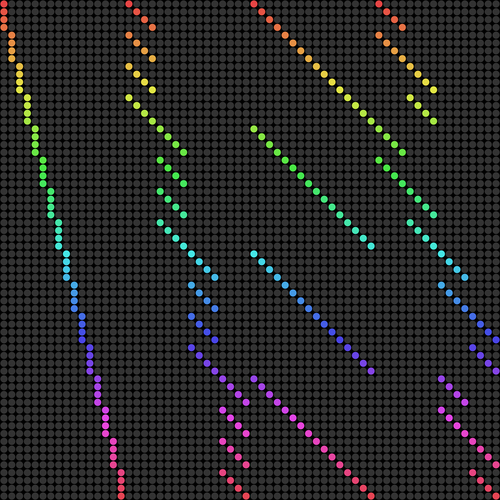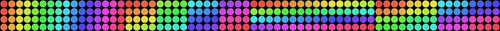# DLX and reduced memory footprint

2012-03-14

The Dancing Links algorithm from Donald Knuth is a well-known method used to solve sudokus (and various other fun things which can be reduced to an exact cover problem). I recently decided to implement it.

For those who aren't familiar with the algorithm, the main idea is to have a huge matrix of all the candidates where columns and "row dependencies" are taken out the matrix in a recursive manner. Knuth proposed a way of removing and restoring these nodes effectively (without a need of any copy).

I won't go into the details of the algorithm and will assume you have basic understanding of it. A lot of people already manage to popularized the method by writing articles about the naive implementation, so you should be able to document yourself enough to get the idea.

The rest of the article will assume the dimension is `D`, and `N=D×D`. For example, a standard sudoku has `D=3` and `N=3×3=9`. Also, the selected language here is the C.

## A huge matrix

If you look at the initial matrix (empty board) of a `D=3` sudoku, it looks like this: Exact Cover Matrix

Each active nodes of the matrix is supposed to be double linked horizontally and vertically to the other active nodes.

So in the matrix you will allocate, each node is supposed to have at least 5 pointers: `left`, `right`, `up`, `down` and the `column`. Also, they most likely have a name to identify them (in order to print the solution). That name can be stored in a char, but with the padding it will likely use more.

On a 32 bits machine, each node will occupy something like 24B, and 48B on 64 bits. The matrix has a size of `N×N×N × N×N×4`, means `9×9×9 × 9×9×4 × 24B = 5668704B`, or more than `5MB`. On 64-bits, you need to multiply per 2. And of course, when you increase the dimension, things get worse. And to be more exact, you also need a row (`N×N×4`) for the column headers, so your matrix size would certainly be `(N×N×N + 1) × N×N×4` if you do not allocate the header separately.

But this can be greatly reduced with a very simple method.

The trick is that the DLX algorithm doesn't require the nodes to be well aligned in the matrix. What matters is how active nodes are linked together (the node selection is based on links and not on the node positions). The dead nodes are never used, so in theory, we can just not allocate them.

Looking at the matrix, you will notice that each column has `N` actives nodes, so you can have an array of `N × N×N×4` nodes. Linking these nodes vertically is pretty simple: you just have to get down vertically in your array. Getting the next node horizontally is slightly more tricky; I personally used the size field in the header to keep track of the last available row (each time a new node is added in the column, the size is incremented).

In the following schema, the nodes with the same color are double-linked together horizontally (matrix with `D=2`):And with the compact matrix I proposed, it looks like this:The horizontal links are still illustrated with the color. For the vertical ones, the default matrix layout is kept (you just have to go up and down).

## Compress me the other way around

Another way of doing this is to reduce the matrix not in height, but in width. While this method might get more intuitive (you have one active node per constraint type, so 4 nodes per row), you might have trouble with the column header. Though, this is certainly possible, and I'd be curious to see such implementation.

## Implementation

Here is the code, see the `init()` function for the matrix initialization. The other functions mostly follow the naming used in the original paper by Knuth so you should be able to get a grip on the code easily.

``````#include <limits.h>
#include <stdint.h>
#include <stdio.h>
#include <stdlib.h>
#include <string.h>

#define D 3
#define N (D*D)

struct node {
union {
char size;      // headers exclusively use size (to find most interesting column)
uint32_t name;  // cells exclusively use name (to print solution)
};
struct node *left, *up, *right, *down;
struct node *column;
};

enum {CST_CELL, CST_ROW, CST_COL, CST_BOX, CST_N};

#define NODES_HEIGHT (N+1) /* +1 for header */
#define NODES_WIDTH  (CST_N * N*N)

struct sudansu {
uint32_t solution[N*N];
struct node h;
struct node nodes[NODES_HEIGHT * NODES_WIDTH];
struct node *rows[N*N*N];
};

static unsigned get_node_position(unsigned type, unsigned r, unsigned c, unsigned z)
{
switch (type) {
case CST_CELL:  return c + r*N;
case CST_ROW:   return z + r*N;
case CST_COL:   return z + c*N;
case CST_BOX:   return z + c/D*N + r/D*D*N;
default: abort();
}
}

#define CELL_NAME(row, col, numchr) ((row)<<16 | (col)<<8 | (numchr))

static void init(struct sudansu *ctx)
{
unsigned i, j, r, c, z, cst_type;
struct node *x, *row_nodes[CST_N]; // one node per line per constraint type

memset(ctx, 0, sizeof(*ctx));

x = ctx->nodes;
for (j = 0; j < NODES_HEIGHT; j++) {
for (i = 0; i < NODES_WIDTH; i++) {
x->up     = j ==              0 ? &ctx->nodes[(NODES_HEIGHT-1)*NODES_WIDTH + i] : x-NODES_WIDTH;
x->down   = j == NODES_HEIGHT-1 ? &ctx->nodes[               0*NODES_WIDTH + i] : x+NODES_WIDTH;
x->column = &ctx->nodes[i];
x++;
}
}

ctx->h.right = ctx->nodes;
ctx->h.left  = ctx->nodes + NODES_WIDTH-1;
for (i = 0; i < NODES_WIDTH; i++) {
ctx->nodes[i].left  = i ==             0 ? &ctx->h : &ctx->nodes[i - 1];
ctx->nodes[i].right = i == NODES_WIDTH-1 ? &ctx->h : &ctx->nodes[i + 1];
}

i = 0;
for (r = 0; r < N; r++) {
for (c = 0; c < N; c++) {
for (z = 0; z < N; z++) {

/* locate the CST_N nodes per row */
for (cst_type = 0; cst_type < CST_N; cst_type++) {
unsigned col = get_node_position(cst_type, r, c, z);
struct node *colhead = &ctx->nodes[cst_type*N*N + col];

// locate first unused node of the colum
x->name = CELL_NAME(r, c, z+'1');
row_nodes[cst_type] = x;
}

for (cst_type = 0; cst_type < CST_N; cst_type++) {
row_nodes[cst_type]->left  = row_nodes[cst_type == 0 ? CST_N-1 : cst_type-1];
row_nodes[cst_type]->right = row_nodes[cst_type == CST_N-1 ? 0 : cst_type+1];
}

/* index the row since the row positions in ctx->nodes are not linear */
ctx->rows[i++] = row_nodes;
}
}
}
}

static void cover(struct node *c)
{
struct node *i, *j;

c->right->left = c->left;
c->left->right = c->right;
for (i = c->down; i != c; i = i->down) {
for (j = i->right; j != i; j = j->right) {
j->down->up = j->up;
j->up->down = j->down;
j->column->size--;
}
}
}

static void uncover(struct node *c)
{
struct node *i, *j;

for (i = c->up; i != c; i = i->up) {
for (j = i->left; j != i; j = j->left) {
j->column->size++;
j->down->up = j->up->down = j;
}
}
c->right->left = c->left->right = c;
}

static int print_solution(const uint32_t *solution)
{
unsigned i, j, k;
char grid[N*N] = {0};
const char *p = grid;

for (i = 0; i < N*N; i++) {
const char row = solution[i]>>16 & 0xff;
const char col = solution[i]>> 8 & 0xff;
const char num = solution[i]     & 0xff;
grid[row*N + col] = num;
}

for (j = 0; j < N; j++) {
for (i = 0; i < N; i++) {
printf(" %c", *p ? *p : '?');
if (i+1 != N && (i+1) % D == 0)
printf(" |");
p++;
}
if (j+1 != N && (j+1) % D == 0) {
printf("\n ");
for (k = 0; k < (N+D-1)*2 - 1; k++)
printf("-");
}
printf("\n");
}
printf("\n");
return 1;
}

static struct node *choose_a_column(struct node *h)
{
int size = INT_MAX;
struct node *j, *c = h->right;

if (c == h)
return NULL;
for (j = h->right; j != h; j = j->right)
if (j->size < size)
c = j, size = j->size;
return c;
}

static int search(struct sudansu *ctx, unsigned k)
{
struct node *c, *r, *j;

c = choose_a_column(&ctx->h);
if (!c)
return print_solution(ctx->solution);
cover(c);
for (r = c->down; r != c; r = r->down) {
ctx->solution[k] = r->name;
for (j = r->right; j != r; j = j->right)
cover(j->column);
if (search(ctx, k + 1))
return 1;
ctx->solution[k] = 0;
for (j = r->left; j != r; j = j->left)
uncover(j->column);
}
uncover(c);
return 0;
}

static int parse_grid(struct sudansu *ctx, FILE *stream)
{
char buf;
unsigned i, j, k = 0;

for (j = 0; j < N; j++) {
if (!fgets(buf, sizeof buf, stream))
return -1;
for (i = 0; i < N; i++) {
if (buf[i] >= '1' && buf[i] <= '1'+N) {
struct node *x, *r = ctx->rows[j*N*N + i*N + buf[i]-'1'];

ctx->solution[k++] = CELL_NAME(j, i, buf[i]);
cover(r->column);
for (x = r->right; x != r; x = x->right)
cover(x->column);
}
}
}
return k;
}

int main(int ac, char **av)
{
int k = 0;
struct sudansu ctx;

init(&ctx);
if (ac == 2 && strcmp(av, "-") == 0) {
k = parse_grid(&ctx, stdin);
if (k < 0)
k = 0;
}
search(&ctx, k);
return 0;
}
``````

For updates and more frequent content you can follow me on Mastodon. Feel also free to subscribe to the RSS in order to be notified of new write-ups. It is also usually possible to reach me through other means (check the footer below). Finally, discussions on some of the articles can sometimes be found on HackerNews, Lobste.rs and Reddit.